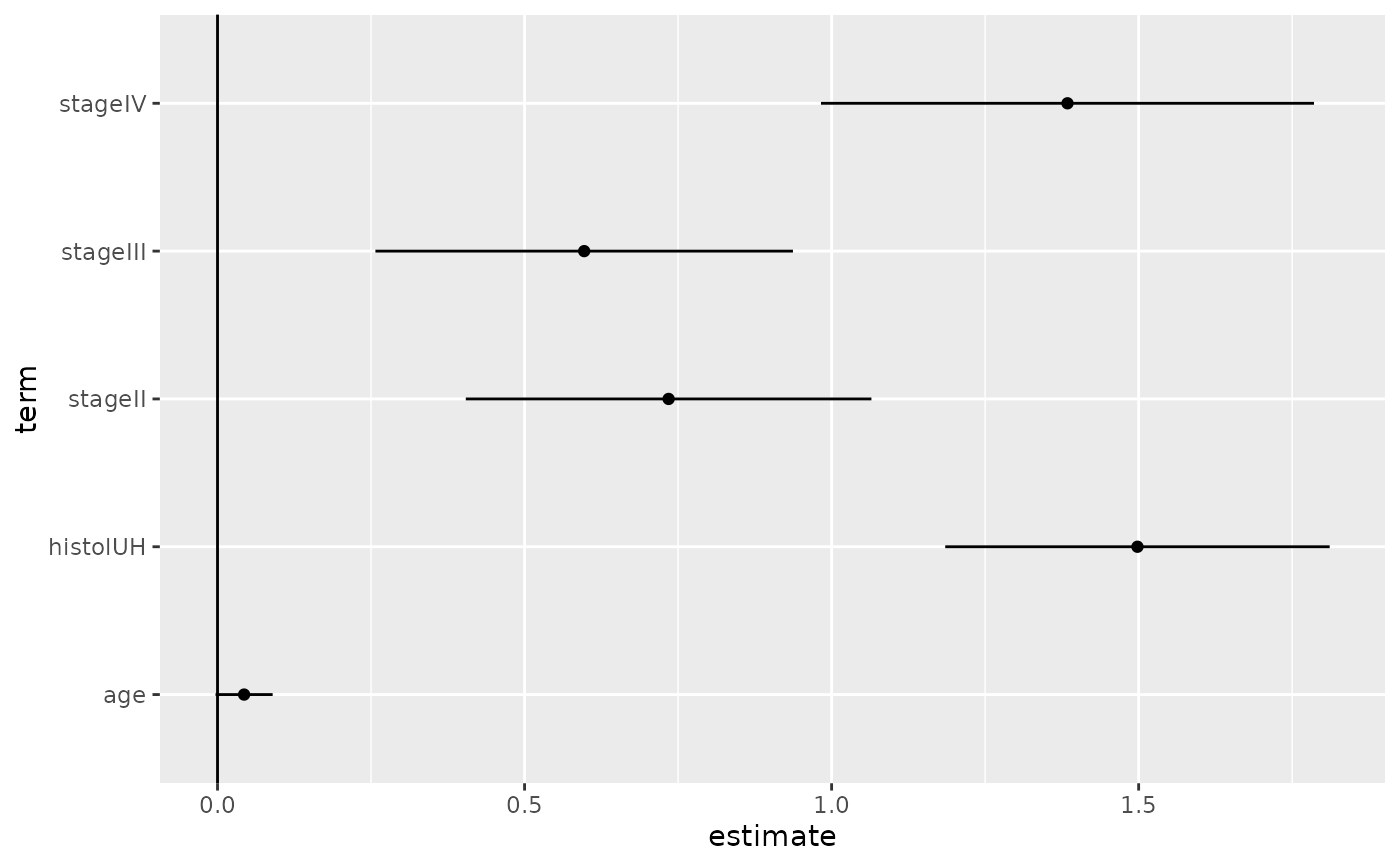Tidy summarizes information about the components of a model. A model component might be a single term in a regression, a single hypothesis, a cluster, or a class. Exactly what tidy considers to be a model component varies across models but is usually self-evident. If a model has several distinct types of components, you will need to specify which components to return.

## Usage

# S3 method for cch
tidy(x, conf.level = 0.95, ...)

## Arguments

x

An cch object returned from survival::cch().

conf.level

confidence level for CI

...

Additional arguments. Not used. Needed to match generic signature only. Cautionary note: Misspelled arguments will be absorbed in ..., where they will be ignored. If the misspelled argument has a default value, the default value will be used. For example, if you pass conf.lvel = 0.9, all computation will proceed using conf.level = 0.95. Two exceptions here are:

• tidy() methods will warn when supplied an exponentiate argument if it will be ignored.

• augment() methods will warn when supplied a newdata argument if it will be ignored.

tidy(), survival::cch()

Other cch tidiers: glance.cch(), glance.survfit()

Other survival tidiers: augment.coxph(), augment.survreg(), glance.aareg(), glance.cch(), glance.coxph(), glance.pyears(), glance.survdiff(), glance.survexp(), glance.survfit(), glance.survreg(), tidy.aareg(), tidy.coxph(), tidy.pyears(), tidy.survdiff(), tidy.survexp(), tidy.survfit(), tidy.survreg()

## Value

A tibble::tibble() with columns:

conf.high

Upper bound on the confidence interval for the estimate.

conf.low

Lower bound on the confidence interval for the estimate.

estimate

The estimated value of the regression term.

p.value

The two-sided p-value associated with the observed statistic.

statistic

The value of a T-statistic to use in a hypothesis that the regression term is non-zero.

std.error

The standard error of the regression term.

term

The name of the regression term.

## Examples


# load libraries for models and data
library(survival)

# examples come from cch documentation
subcoh <- nwtco$in.subcohort selccoh <- with(nwtco, rel == 1 | subcoh == 1) ccoh.data <- nwtco[selccoh, ] ccoh.data$subcohort <- subcoh[selccoh]

# central-lab histology
ccoh.data$histol <- factor(ccoh.data$histol, labels = c("FH", "UH"))

# tumour stage
ccoh.data$stage <- factor(ccoh.data$stage, labels = c("I", "II", "III", "IV"))
ccoh.data$age <- ccoh.data$age / 12 # age in years

# fit model
fit.ccP <- cch(Surv(edrel, rel) ~ stage + histol + age,
data = ccoh.data,
subcoh = ~subcohort, id = ~seqno, cohort.size = 4028
)

# summarize model fit with tidiers + visualization
tidy(fit.ccP)
#> # A tibble: 5 × 7
#>   term     estimate std.error statistic  p.value conf.low conf.high
#>   <chr>       <dbl>     <dbl>     <dbl>    <dbl>    <dbl>     <dbl>
#> 1 stageII    0.735     0.168       4.36 1.30e- 5  0.404      1.06
#> 2 stageIII   0.597     0.173       3.44 5.77e- 4  0.257      0.937
#> 3 stageIV    1.38      0.205       6.76 1.40e-11  0.983      1.79
#> 4 histolUH   1.50      0.160       9.38 0         1.19       1.81
#> 5 age        0.0433    0.0237      1.82 6.83e- 2 -0.00324    0.0898

# coefficient plot
library(ggplot2)

ggplot(tidy(fit.ccP), aes(x = estimate, y = term)) +
geom_point() +
geom_errorbarh(aes(xmin = conf.low, xmax = conf.high), height = 0) +
geom_vline(xintercept = 0)